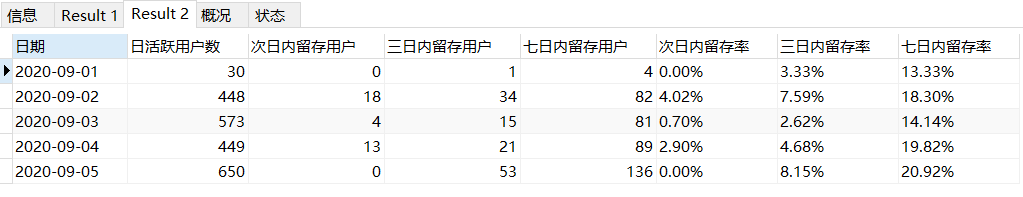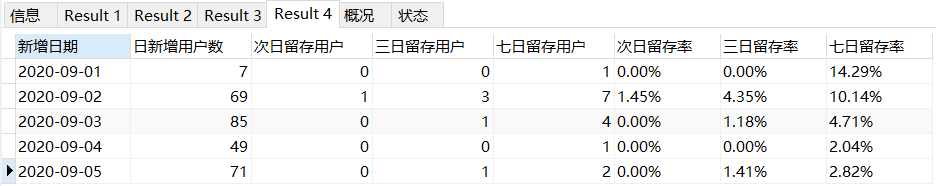# Mysql查询用户留存/留存率语法

• 计算某日的客户在第n日再次出现的概率--用户n日留存率。

• 计算某日的客户在某个时间段内再次出现的概率--用户n日内留存率。

• 计算某日新增的用户在第n日再次出现的概率--新用户n日留存率。

• 计算某日新增的用户在某个时间段内再次出现的概率--新用户n日内留存率。

## 1.用户n日留存/留存率

• 表名:订单总表;字段:客户编号,下单时间

``````-- 1.用户n日留存/留存率
-- 用户次日、3日、7日、30日,...留存/留存率

select *,
concat(round(100*次日留存用户/日活跃用户数,2),'%')  次日留存率,
concat(round(100*三日留存用户/日活跃用户数,2),'%')  三日留存率,
concat(round(100*七日留存用户/日活跃用户数,2),'%')  七日留存率
from (
select
date(a.下单时间) as 日期,
count(distinct a.客户编号) as 日活跃用户数,
count(distinct b.客户编号) as 次日留存用户,
count(distinct c.客户编号) as 三日留存用户,
count(distinct d.客户编号) as 七日留存用户
from 订单总表 a
left join 订单总表 b
on a.客户编号 = b.客户编号
and date(b.下单时间) = date(a.下单时间) + 1
left join 订单总表 c
on a.客户编号 = c.客户编号
and date(c.下单时间) = date(a.下单时间) + 3
left join 订单总表 d
on a.客户编号 = d.客户编号
and date(d.下单时间) = date(a.下单时间) + 7
where date(a.下单时间) between "2020/09/01" and "2020/09/05"
group by date(a.下单时间)
) p;
``````## 2.用户n日内留存/留存率

``````-- 2.用户n日内留存/留存率
-- 用户次日内、3日内、7日内、30日内,...留存/留存率

select *,
concat(round(100*次日内留存用户/日活跃用户数,2),'%')  次日内留存率,
concat(round(100*三日内留存用户/日活跃用户数,2),'%')  三日内留存率,
concat(round(100*七日内留存用户/日活跃用户数,2),'%')  七日内留存率
from (
select
date(a.下单时间) as 日期,
count(distinct a.客户编号) as 日活跃用户数,
count(distinct b.客户编号) as 次日内留存用户,
count(distinct c.客户编号) as 三日内留存用户,
count(distinct d.客户编号) as 七日内留存用户
from 订单总表 a
left join 订单总表 b
on a.客户编号 = b.客户编号
and date(b.下单时间) = date(a.下单时间) + 1
left join 订单总表 c
on a.客户编号 = c.客户编号
and (date(c.下单时间) BETWEEN date(a.下单时间) + 1 AND date(a.下单时间) + 3)
left join 订单总表 d
on a.客户编号 = d.客户编号
and (date(d.下单时间) BETWEEN date(a.下单时间) + 1 AND date(a.下单时间) + 7)
where date(a.下单时间) between "2020/09/01" and "2020/09/05"
group by date(a.下单时间)
) p;
``````## 3.新增用户n日留存/留存率

``````## 3.某日新增用户留存/留存率
## 计算某日新增的用户在次日、3日、7日的留存率--新用户n日留存

select *,
concat(round(100*次日留存用户/日新增用户数,2),'%')  次日留存率,
concat(round(100*三日留存用户/日新增用户数,2),'%')  三日留存率,
concat(round(100*七日留存用户/日新增用户数,2),'%')  七日留存率
from (
select
date(a.下单时间) as 新增日期,
count(distinct a.客户编号) as 日新增用户数,
count(distinct b.客户编号) as 次日留存用户,
count(distinct c.客户编号) as 三日留存用户,
count(distinct d.客户编号) as 七日留存用户
from
(
select
date(t1.下单时间) as 下单时间,
t1.客户编号
from 订单总表 as t1
left join 订单总表 as t2
on t1.客户编号=t2.客户编号
and t2.下单时间<t1.下单时间
where
(date(t1.下单时间) between "2020/09/01" and "2020/09/05")
and t2.下单时间 is Null
) as a
left join 订单总表 b
on a.客户编号 = b.客户编号
and date(b.下单时间) = date(a.下单时间) + 1
left join 订单总表 c
on a.客户编号 = c.客户编号
and date(c.下单时间) = date(a.下单时间) + 3
left join 订单总表 d
on a.客户编号 = d.客户编号
and date(d.下单时间) = date(a.下单时间) + 7
group by date(a.下单时间)
) p;
``````## 4.新增用户n日内留存率

``````## 4.新增用户n日内留存率
## 计算某日新增的用户在次日内、3日内、7日内的留存率--新用户n日内留存率。
select *,
concat(round(100*次日留存用户/日新增用户数,2),'%')  次日留存率,
concat(round(100*三日留存用户/日新增用户数,2),'%')  三日留存率,
concat(round(100*七日留存用户/日新增用户数,2),'%')  七日留存率
from (
select
date(a.下单时间) as 新增日期,
count(distinct a.客户编号) as 日新增用户数,
count(distinct b.客户编号) as 次日留存用户,
count(distinct c.客户编号) as 三日留存用户,
count(distinct d.客户编号) as 七日留存用户
from
(
select
date(t1.下单时间) as 下单时间,
t1.客户编号
from 订单总表 as t1
left join 订单总表 as t2
on t1.客户编号=t2.客户编号
and t2.下单时间<t1.下单时间
where
(date(t1.下单时间) between "2020/09/01" and "2020/09/05")
and t2.下单时间 is Null
) as a
left join 订单总表 b
on a.客户编号 = b.客户编号
and date(b.下单时间) = date(a.下单时间) + 1
left join 订单总表 c
on a.客户编号 = c.客户编号
and (date(c.下单时间) BETWEEN date(a.下单时间) + 1 AND date(a.下单时间) + 3)
left join 订单总表 d
on a.客户编号 = d.客户编号
and (date(d.下单时间) BETWEEN date(a.下单时间) + 1 AND date(a.下单时间) + 7)
group by date(a.下单时间)
) p;
``````### 如何同时安装jdk7和jdk8_jdk8安装后在安装jdk7_weixin_44696786的博客-程序员秘密

jdk1.7和jdk1.8同时安装

### PaperNotes(4)-高质量图像生成-CGAN-StackGAN-Lapgan-Cyclegan-Pix2pixgan_cgan图像生成_小陈同学-陈百万的博客-程序员秘密

cgan,stackgan,lapgan,cyclegan,pix2pixgan简记1.Conditional GAN1.1简介1.2网络结构与训练1.3特点与用途2.Stack GAN2.1简介2.2网络结构与训练2.3特点与用途3.Lap GAN3.1简介3.2网络结构与训练3.3特点与用途4.Pix2pix GAN4.1 简介4.2 网络结构和训练4.3 特点和用途5.Patch GAN6.Cycle GAN6.1简介6.2网络结构与训练6.3特点与用途7.思考与待定题记–如何生成高质量图像，gan

### openGauss内核荣获中国首个国际CC EAL4+级别认证_opengauss社区认证_openGauss社区的博客-程序员秘密

openGauss内核荣获中国首个国际CC EAL4+级别认证

### CANoe中使用CAPL函数接口调用Vflash文件_蚂蚁小兵的博客-程序员秘密

CANoe中使用CAPL函数接口调用Vflash文件

### RAID的几种工作模式_raid6的工作方式_她与星辰皆璀璨的博客-程序员秘密

**RAID的几种工作模式** 1、RAID0即Data Stripping数据分条技术。RAID 0可以把多块硬盘连成一个容量更大的硬盘群，可以提高磁盘的性能和吞吐量。RAID 0没有冗余或错误修复能力，成本低，要求至少两个磁盘，一般只是在那些对数据安全性要求不高的情况下才被使用。（1）、RAID ...

### Netty的入门-UDP协议开发_closefuture().await();_Eric_LanOu的博客-程序员秘密

User Datagram Protocal直接用IP协议进行数据发送，提供的是面向无连接，不可靠的数据投递服务。使用UDP的应用程序必须自己解决数据丢失，重复，排序，差错确认等问题。